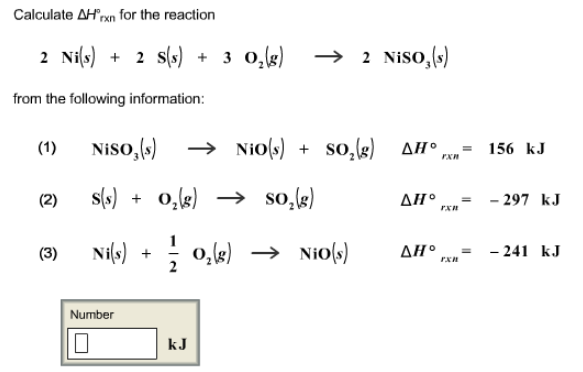# Problem: Calculate ΔH°rxn for the reaction 2Ni (s) + 2S (s) + 3O2 (g) → 2NiSO3 (s) from the following information: (1) NiSO3 (s) → NiO (s) + SO3 (g)     ΔH°rxn = 156 kJ (2) S (s) + O2 (g) → SO2 (g)     ΔH°rxn = - 297 kJ (3) Ni (s) + 1/2O2 (g) → NiO (s)     ΔH°rxn = -241 kJ

🤓 Based on our data, we think this question is relevant for Professor Pelton's class at UMN.

###### FREE Expert Solution###### Problem Details

Calculate ΔH°rxn for the reaction

2Ni (s) + 2S (s) + 3O2 (g) → 2NiSO3 (s)

from the following information:

(1) NiSO3 (s) → NiO (s) + SO3 (g)     ΔH°rxn = 156 kJ

(2) S (s) + O2 (g) → SO2 (g)     ΔH°rxn = - 297 kJ

(3) Ni (s) + 1/2O2 (g) → NiO (s)     ΔH°rxn = -241 kJ